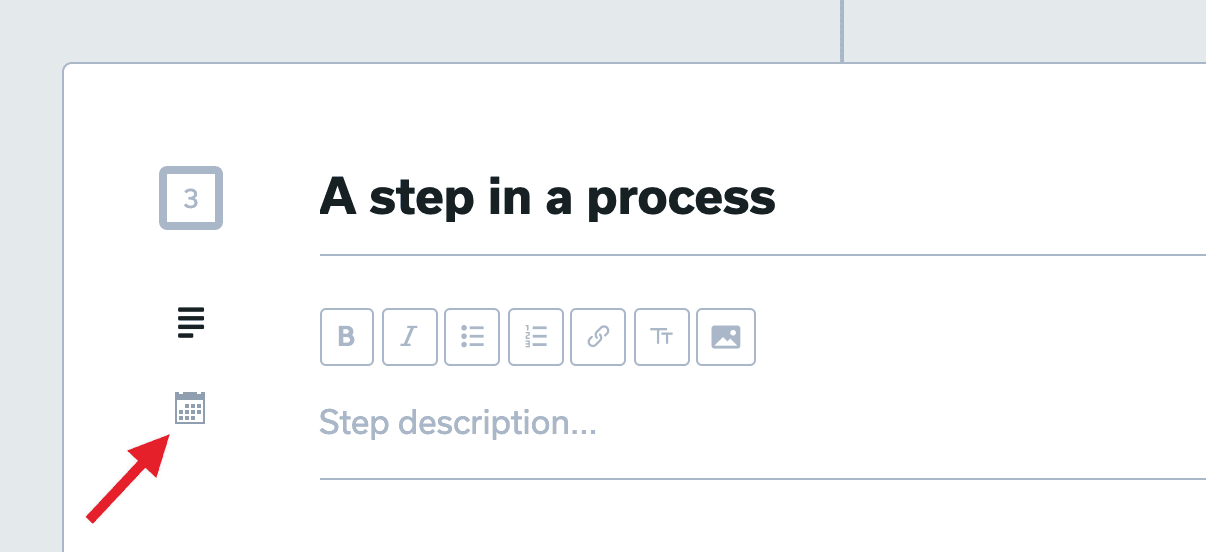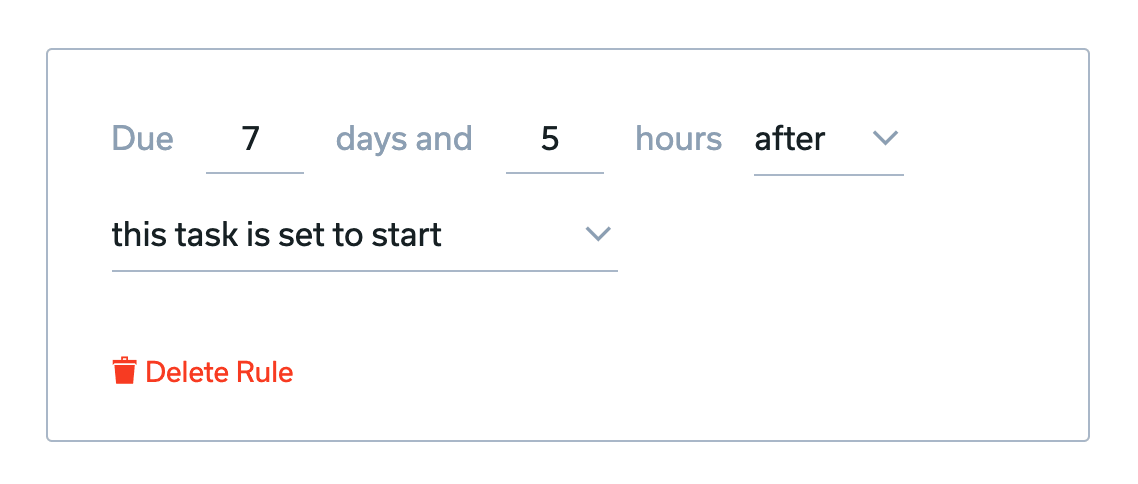# Creating dynamic start & due date rules on steps in a process

When editing a process template, you can define "date rules" on each step to say how its start or due date should be calculated once this process is used on a project and those steps convert into tasks.  By defining these date rules, your process can easily be re-used many times on your projects, each time with new dates calculated and automatically applied.

Most commonly, date rules are created in your processes, so that they can be re-used many times in the future (when your process is copied to a task list on a project).  But you can also create and manage date rules on individual tasks on the project level too.

## Create date rules on steps in a process

1. Create or edit a process template and create or edit some step(s) in this process.
2. Click the calendar icon to reveal the date rules section on the step.3. Click "Add a start date rule" or "Add a due date rule"
4. Fill in the details on the rule (more on this below)
5. Click the "Update" button on the step to save the rule.

## Understanding date rule details• Every date rule must have a number of days or a number of hours set (or both).  You can define "0" in either of these as well.
• You must set "before" or "after"
• You must set the rule criteria...

### Rule criteria options:

• "the project is set to start"
This will calculate the date relative to the project's start date. If the the project doesn't have its start date set, then this rule will use the date the project was created instead.
• "the project is set to end"
The date will be calculated based on the project's end date.  If the project's end date is not set, then this rule will be ignored.
• "this task is set to start/is due"
If this rule is for the start date, then it will be calculated based on this task's due date.  If the rule is for the due date, then it will calculate based on this task's start date.  If the start or due date isn't set, then this rule will be ignored.
When this criteria is selected, then it reveals an additional dropdown where you must select which other step in the process to reference.  Assuming that other task has a due date, then this task's date will be calculated in relation to that.  If that other step doesn't have a due date, then this rule will be ignored.
When this criteria is selected, then it reveals an additional dropdown where you must select which other step in the process to reference.  At the time that other task is marked completed, this task's start/due date will be calculated in relation to that.